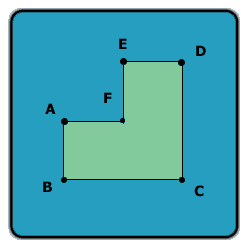All about flooble | fun stuff | Get a free chatterbox | Free JavaScript | Avatarsperplexus dot infoA different angle (Posted on 2003-10-10)Look at this shape:Assume AB = AF = FE = ED and BC = CD, and all the angles in the shape are 90 degrees.

Let A be the area of this shape (in cm^2), and P -- the perimeter of this shape (in cm).

If A - P is 60, what is the length of line AC?

 See The Solution Submitted by Lewis Rating: 1.9167 (12 votes)Comments: ( Back to comment list | You must be logged in to post comments.)Puzzle Solution With Explanation Comment 23 of 23 |Let the sides BC =CD= y(say)
Then, A = y^2 -(y^2/4) = 3*(y^/4)
and, P = 2y+2y = 4y

Thus, by conditions of the problem:

3*(y^2/4) - 4y = 60
Or, 3*y^2 - 16y -240 = 0
Or, (y-12)(3y+ 20) = 0
Or, y = 12, ignoring the negative root which is inadmissible.

Consequently, AC = V(y^2 + (y^2)/4)
= V(6^2+12^2)
=  13.4164 units (approx.)

Edited on September 25, 2007, 1:38 pm
 Posted by K Sengupta on 2007-05-20 15:16:30Please log in:

 Search: Search body:
Forums (5)